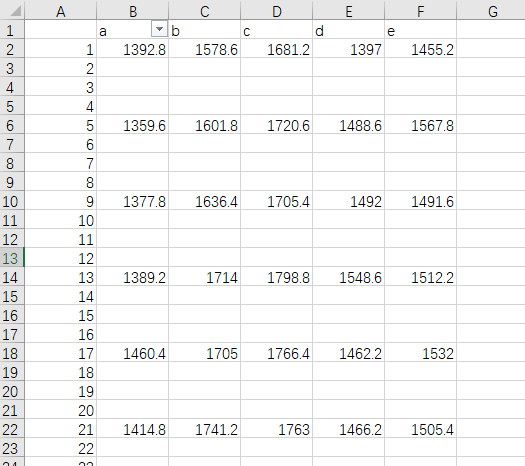``````import pandas as pd
from scipy.interpolate import lagrange
inputfile = './test1.xlsx'
outputfile = './test1_1.xlsx'
def ployinterp_column(s,n,k=6):
y = s[list(range(n-k,n)) + list(range(n+1,n+1-k))]
y = y[y.notnull()]
return lagrange(y.index,list(y))(n)
for i in data.columns:
for j in range(len(data)):
if (data[i].isnull())[j]:
data[i][j] = ployinterp_column(data[i],j)
``````

``````KeyError                                  Traceback (most recent call last)
<ipython-input-28-02cf873cddff> in <module>()
1 for i in data.columns:
2     for j in range(len(data)):
----> 3         if (data[i].isnull())[j]:
4             if (data[i].isnull())[j] in data:
5                 data[i][j] = ployinterp_column(data[i],j)

C:\Anaconda3\lib\site-packages\pandas\core\series.py in __getitem__(self, key)
765         key = com._apply_if_callable(key, self)
766         try:
--> 767             result = self.index.get_value(self, key)
768
769             if not is_scalar(result):

C:\Anaconda3\lib\site-packages\pandas\core\indexes\base.py in get_value(self, series, key)
3116         try:
3117             return self._engine.get_value(s, k,
-> 3118                                           tz=getattr(series.dtype, 'tz', None))
3119         except KeyError as e1:
3120             if len(self) > 0 and self.inferred_type in ['integer', 'boolean']:

pandas\_libs\index.pyx in pandas._libs.index.IndexEngine.get_value()

pandas\_libs\index.pyx in pandas._libs.index.IndexEngine.get_value()

pandas\_libs\index.pyx in pandas._libs.index.IndexEngine.get_loc()

pandas\_libs\hashtable_class_helper.pxi in pandas._libs.hashtable.Int64HashTable.get_item()

pandas\_libs\hashtable_class_helper.pxi in pandas._libs.hashtable.Int64HashTable.get_item()

KeyError: 0

``````